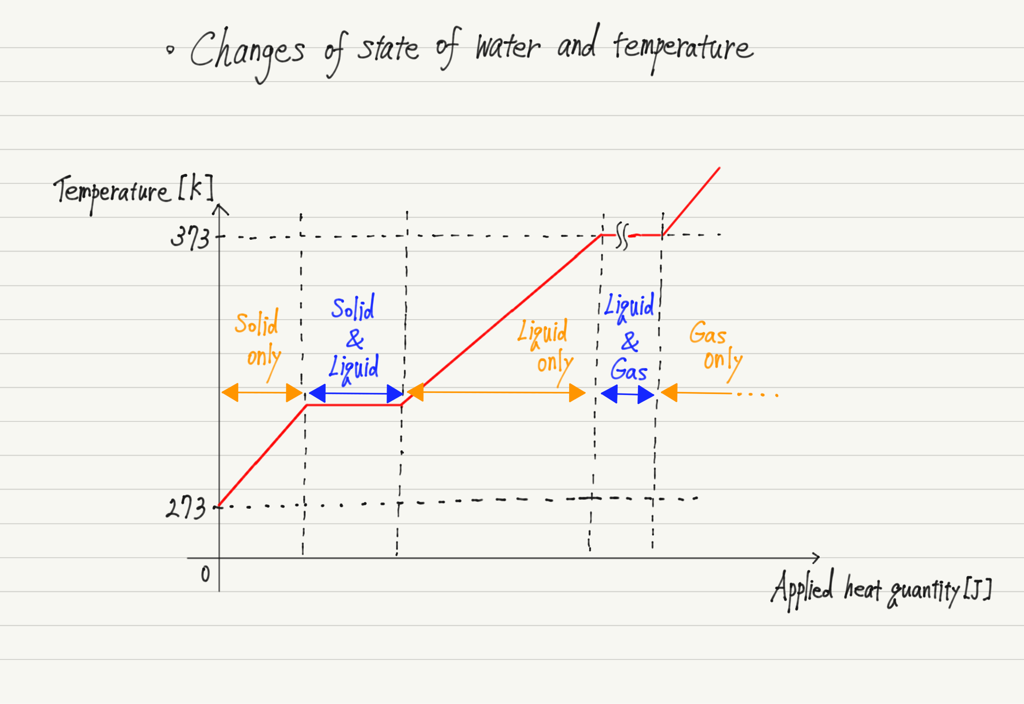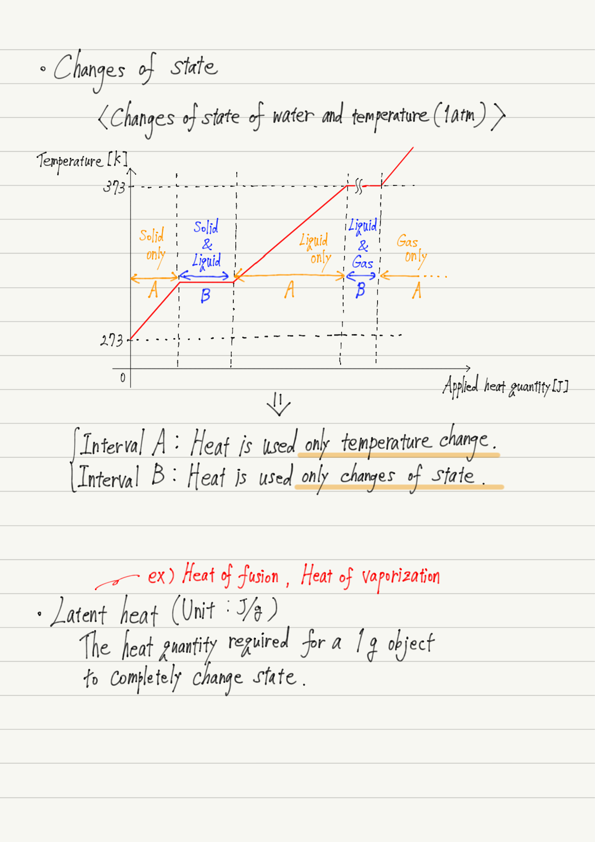# States of Matter and Latent Heat

(This article was translated from Japanese to English using DeepL.)

Now that you have learned about specific heat and heat capacity, you can calculate the change in temperature when heat is applied to an object.

However, we know that “water boils at 273 K(=0 °C=32 °F), and the temperature remains at 373 K(=100 °C=212 °F) even if we continue to heat it.

What does it mean that the temperature does not change when heat is applied?
In this lecture we will learn about the relationship between heat and temperature rise.

## Changes of state

Matter has three basic states: solid, liquid, and gas, and pressure and temperature determine which of these states it will be in (let’s fix the pressure at 1 atm for the present discussion).

Standard materials change from solid to liquid and liquid to gas when heat is applied.
(Some substances, such as carbon dioxide, iodine, and naphthalene, can be converted directly from solid to gas.)
Molecules tightly bound together were solids, loosened bonds were liquids, and disconnected bonds were gases.

## Graph of temperature change

Let’s take water, the most familiar substance, as an example to see how temperature changes.The key to this graph is “what was the applied heat used for?”
Heat can be used in two ways.
1. Heat used to intensify the thermal motion of molecules.
2. Heat used to break the bonds between molecules.
For the usage 1, the temperature rises, and for the usage 2, a change of states occurs.

## How heat is used in matter

Let us look again at the previous graph.

Even if ice is heated, it does not melt until it reaches 273 K (solid state only).
However, when the temperature reaches 273 K, it does not rise again until all of it is melted.

Similarly, liquid water does not boil until it reaches 373 K (liquid state only). However, once it reaches 373 K, the temperature does not rise until it has all evaporated.

This indicates that the temperature rise and the changes of state do not occur simultaneously.

This means that all the applied heat will be used for either usage 1 or 2!
(It is impossible to use part of the heat in 1 and the rest in 2.)

## Latent heat

Last but not least, the heat used for the usage 2 above is called latent heat, and is defined as the amount of heat required for 1 g of a substance to completely change state.

The main latent heat is the heat of fusion and vaporization.
By definition, the heat of fusion is the heat quantity required for 1 g of solid to become completely liquid, while the heat of vaporization is the heat quantity required for 1 g of liquid to become completely gaseous.

## Summary of this lecture## Next Time

I would like to introduce an important law in thermodynamics.

https://www.yukimura-physics.com/entry/therm-f07

error: Content is protected !!